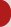English on the Internet - Useful English## Numerals, Calculations

 1 one 21 twenty-one 2 two 22 twenty-two 3 three 23 twenty-three 4 four 30 thirty 5 five 40 forty 6 six 50 fifty 7 seven 60 sixty 8 eight 70 seventy 9 nine 80 eighty 10 ten 90 ninety 11 eleven 100 a hundred 12 twelve 101 one hundred and one 13 thirteen 102 one hundred and two 14 fourteen 200 two hundred 15 fifteen 700 seven hundred 16 sixteen 900 nine hundred 17 seventeen 1000 a thousand 18 eighteen 7000 seven thousand 19 nineteen 1 000 000 a million 20 twenty 3 752 three thousand seven hundred and fifty-two

 0 zero, naught, nil, love 1 000 000 000 (GB) milliard 1 000 000 000 (USA) billion

Fractions - Zlomky
1/2 = one half (of the tax)
1/3 = a / one third (of the income)
1/4 = a / one quarter
1/5 = a / one fifth
5/8 = five eighths
112/213 = a hundred and twelve over two hundred and thirteen

Decimal numbers - Desetinná čísla
0.29 = (nought) point twenty-nine (GB); zero point twenty-nine
4.55 = four point fifty-five

## Calculations - Početní úkony

8 + 7 = 15
What's eight and / plus seven? Eight and / plus seven is / are fifteen.
811 + 131 = 944
Eight hundred and eleven plus a hundred and thirty-one is / equals nine hundred and forty-four.

Subtraction - Odčítání
8 - 3 = 5
Eight from three leaves / is five.
518 - 347 = 171
Five hundred and eighteen minus three hundred and forty-seven is / equals a hundred and seventy-one.

Multiplication - Násobení
18 x 256 = 4608
Eighteen times / multiplied by two hundred and fifty-six is / makes / equals four thousand six hundred and eight.

Division - Dělení
264 : 8 = 33
Two hundred and sixty-four divided by eight is / equals thirty-three.

Raising to a power - Umocňování
52 = 25
Five squared is / equals twenty-five.
53 = 125
Five cubed is / equals a hundred and twenty-five.
56 = 15 625
Five to the sixth (power) is / equals fifteen thousand six hundred and twenty-five.

Extraction of a root - Odmocňování
The square root of nine is / equals three.
The cube root of twenty-seven is / equals three.

© October 1999 English on the Internet www.aj.cz
english@centrum.cz